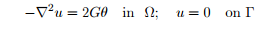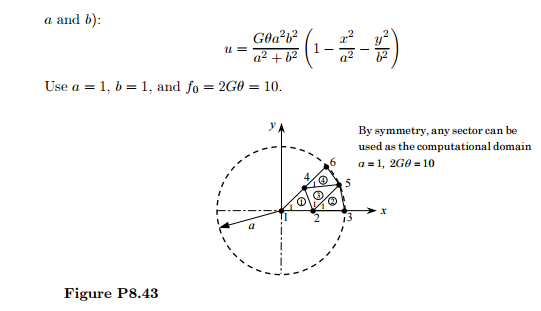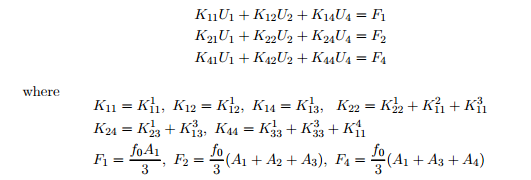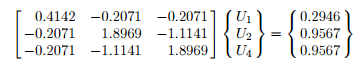# The Prandtl Theory Of Torsion Of A Cylindrical Member Leads To Where Is The Cross Se 2212707

The Prandtl theory of torsion of a cylindrical member leads towhere Ω is the cross section of the cylindrical member being twisted, Γ is the boundary of Ω, G is the shear modulus of the material of the member, θ is the angle of twist, and u is the stress function. Solve the equation for the case in which Ω is a circular section (see Fig. P8.43) using the mesh of linear triangular elements. Compare the finite-element solution with the exact solution (valid for elliptical sections with axessol4

For the mesh shown in a quadrant, the specified degrees of freedom are: U3 = U5 = U6 = 0, and the values at nodes 1, 2 and 4 are to be determined. The condensed equations areand Ai is the area of the ith element and f0 = 2Gθ = 10. The condensed equations are given byThe solution of these equations is: U1 = 2.6292, U2 = 1.9179, U4 = 1.9179. The exact solution at these is given by u1 = 2.5, u2 = 1.875, u4 = 1.875.4.6/5

Price (USD)
\$